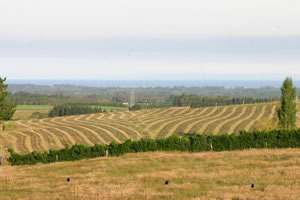# A couple of easy dry matter tests

November 10, 2012
Share:So you don’t have a pasture probe or a rising plate meter but you want to know how many kgs of dry
matter per hectare you have? Or you want to know what the moisture content of your grass is?

Here are some quick techniques for working it out.

The microwave method

Cut a 50cm x 50cm patch of grass as low as you can removing all dead materials.

Multiply the weight of your fresh sample by 40 e.g. 200g x 40 = 8000kg fresh material per hectare.

Then weigh out 100g of this grass, chop it up and then put it on a paper plate in the microwave.

Then microwave it for four to five minutes. Remove the grass and weigh it.

Then put it back on the paper plate and microwave it for another two minutes. Remove it from the
microwave again and weigh it. Continue to microwave it for two minutes at a time and then weigh it until
there is no change in weight.

Calculate the dry matter proportion by dividing the final weight by the weight of the fresh material e.g.
35g divided by 100g = 0.35 Then subtract 0.1 (because the microwave does not get the sample totally dry).
This gives you 0.25 or 25% DM.

Multiply the fresh weight per hectare by your final dry matter percentage, e.g. 8000kg fresh material x
0.25 = 2000kgDM/ha.

Add 400g kgDM/ha to account for the grass below your cutting level. In this example it gives you:
2400kgDM/ha

The microwave moisture test

The moisture content is the difference between the original weight of the sample and the weight after
microwaving. i.e. the above sample is 25% dry matter and 75% moisture.

The oven test

Very similar to the microwave test except that you spread the 100g of grass on to an oven tray and dry in
the oven at 100degC for 24 hours. You then do the same calculation as above but you do not have to allow
the 0.1 (for the microwave).

Category: Feed and Fert### About the Author (Author Profile)

1.Robin says: